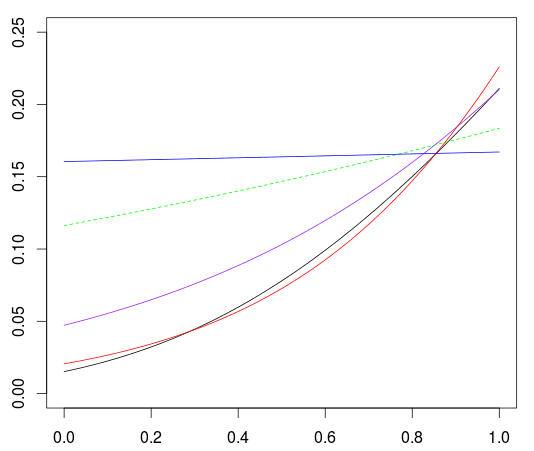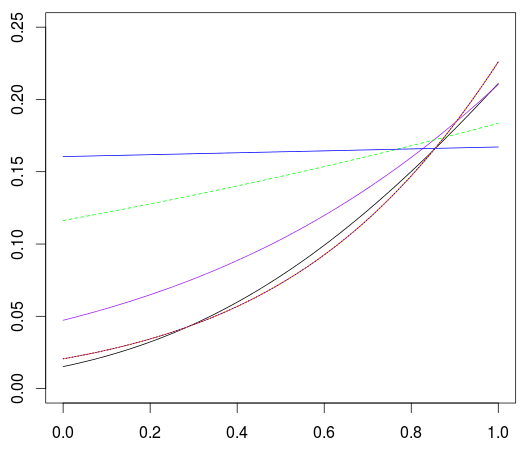Want to share your content on R-bloggers? click here if you have a blog, or here if you don't.

This afternoon, an interesting point was raised, and I wanted to get back on it (since I did publish a post on that same topic a long time ago). How can we adapt a logistic regression when all the observations do not have the same exposure. Here the model is the following: ,

• the occurence of an event  on the period  is unobserved
• the occurence of an event  on  is observed (as well as )

If we assume that the ‘occurence of an event’ is the first occurence of a Poisson processus, we can prove that

i.e. no event occur on  if no event occur on  and no event occur on . Assuming independence between the two, we can prove that we have

With words, it means that the probability of not having a claim in the first six months of the year is the square root of not have a claim over a year. Which makes sense.

Let us generate some sample to play with it, and see if we can use some regression model here.

```> n=1e5
> set.seed(1)
> X = runif(n)
> N = rexp(n,.2)
> E = runif(n,X)
> Y = (N<E)*1
> df=data.frame(Y,E=ceiling(E*12)/12,X)
> tail(df)
Y         E          X
99995  0 0.8333333 0.39316275
99996  0 0.9166667 0.03091459
99997  0 1.0000000 0.22474944
99998  0 1.0000000 0.98475575
99999  0 0.5000000 0.09235741
100000 0 0.2500000 0.12311414```

Here, the average exposure if almost 80% of a year,

```> mean(df\$E)
 0.7883208```

and 15% of the observation experienced an event

```> mean(df\$Y)
 0.14029```

Assume that the probability of not observing an event can be explained by some covariates, denoted  through some link function (using the GLM terminology),

Now, since we do observe  – and not  – we have

So if use a log link function, we can have the same property as in the Poisson model, and the logarithm of the exposure should be taken as an offset variable.

```> reg0=glm((Y==0)~X+offset(log(X)),data=df,
```

But we get an error here, since no starting values for the algorithm could be found. If we try to set them, we get the same error, e.g.

```> reg_init=glm((Y==0)~X+offset(log(X)),data=df,
> reg0=glm((Y==0)~X+offset(log(X)),data=df,

So, let us try another model. An alternative could be to use

which is the so-called complementary log-log link, cloglogWe can also write the inverse of that link function, which remins us Gompertz distribution,

Observe that

which can also be written

and therefore, one can prove that

So here we can, as in the Poisson case, add the logarithm of the exposure as an offset variable,

```> reg1=glm((Y==0)~X+offset(log(E)),

and the prediction of the probability to observe an event (over a full year) is

```> vx=seq(0,1,by=.01)
> v1=1-predict(reg1,newdata=data.frame(X=vx,E=1),
type="response")```

That is nice, but what if we want to use a probit transformation for the annual probability? Something like

I’ve seen people trying either

```> reg2=glm((Y==0)~X+offset(log(E)),data=df,
family=binomial)```

or

```> reg3=glm((Y==0)~X,weight=E,data=df,
family=binomial)```

But it does not really makes sense. Actually, if we plot those three predictions, we get

```> plot(vx,v1)
> v2=1-predict(reg2,newdata=data.frame(X=vx,E=1),
type="response")
> lines(vx,v2,col="purple")
> v3=1-predict(reg3,newdata=data.frame(X=vx,E=1),
type="response")
> lines(vx,v3,col="green",lty=2)```How can we get something better? A first idea could to actually compute the log-likelihood, and to optimize it. Manually,

```> Y=(df\$Y==1)*1
>  X=cbind(1,df\$X)
>  E=df\$E
>  logL = function(beta){
+    pi=(exp(X%*%beta)/(1+exp(X%*%beta)))^E
+   	-sum(log(dbinom(Y,size=1,prob=pi)))
+   }
>  P=optim(fn=logL,par=c(-0.0001,-.001),
+      method="BFGS")```

From that optimization, we can also compute a prediction

```>  beta=P\$par
>  v4 = exp(beta+beta*vx)/
(1+exp(beta+beta*vx))```

Another strategy can actually to work not on a yearly basis, but on a monthly basis. If for someone observed one month, there were no event, we should have one line, and a 0. If for someone observed three month, there was one event, we should have three lines (one per month) and two 0’s, and one 1. So first, we have to generate the monthly dataset,

```> rep_each=function(x,n){
+   y=NULL
+   for(i in 1:length(n)) y=c(y,rep(x[i],n[i]))
+   return(y)
+ }
> split_freq=function(Y,E){
+   y=NULL
+   for(i in 1:length(Y)){
+     if(12*E[i]>1) v=c(Y[i],rep(0,12*E[i]-1))
+     if(12*E[i]==1) v=Y[i]
+     y=c(y,v)
+   }
+   return(y)
+ }

> index=rep_each(1:n,df\$E*12)
> large_df=df[index,]
> large_df\$Y=split_freq(df\$Y,df\$E)```

In the initial dataset, we observed 14,000 events,

```> sum(df\$Y)
 14029```

and here also

```> sum(large_df\$Y)
 14029```

But this time, there are much more raws: one per month of observation. We can now run a ‘standard’ logistic regression to predict the monthly probability to observe an event

`> reg5=glm(Y~X,family=binomial,data=large_df)`

and then get a yearly prediction

```> v5=predict(reg5,newdata=data.frame(X=vx),
type="response")
> v5_year=1-(1-v5)^12```

But it is not really working here

```> lines(vx,v4,col="red")
> lines(vx,v5_year,col="blue")```(there was already a post on this idea of replicating data, in the context of contingency table, trying to make connections between weights and frequency).

As mentioned on rstudio-pubs-static, and discussed in journal.frontiersin.org, one can also actually use an R package dedicated to this problem,

```> library(bbmle)
> reg6 <- mle2(Y~dbinom(plogis(mu)^E,size=1),
+   parameters=list(mu~X),
+   start=list(mu=2),data=df)```

Our prediction is here

```> v6=predict(reg6,newdata=data.frame(X=vx,E=1),
type="response")```

Last, but not least, it is also possible to use Shaffer’s method (see raw.githubusercontent.com/zhenglei-gao/ for some code),

```> library(MASS)
> logexp <- function(exposure = 1)
+ {
+ logit_mu_eta <- function(eta) {
+     ifelse(abs(eta)>30,.Machine\$double.eps,
+            exp(eta)/(1+exp(eta))^2)
+   }
+   mu.eta <- function(eta) {
+     exposure * plogis(eta)^(exposure-1) *
+     logit_mu_eta(eta)
+   }
+   valideta <- function(eta) TRUE
+   link <- paste("logexp(", deparse(substitute(exposure)), ")", sep="")
+ }

> reg7 <- glm(Y~X,
+ data=df,start=c(1,0))```

The main problem with this method is that the exposure is not a variable name, but a vector, and we can only get a prediction for observation in the dataset. But it is possible here to get a lot of predictions, and to plot them,

```> v7=predict(reg7,type="response")
> d=data.frame(x=df\$X,y=v7,e=df\$E)
> d=d[d\$e==1,]
> d=d[order(d\$x),]
> lines(vx,v7,col="orange",lty=2)
> lines(d\$x,d\$y,col="blue",lty=3)```Here, we have various techniques that can be used to take into account the exposure in a logistic regression. Three of them are them are identical (numerical optimization, bbmle and Shaffer), one is close (the complementary log-log) and the other three are very far away (including weights and using in a standard logistic regression the offset component).